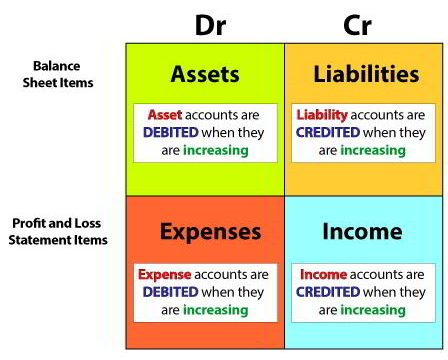# Debit and Credit in Accounting Double Entry SystemEvery transaction affects the accounting equation of a business. Dual change may take place between two assets.

Accounts must be appropriately credited and debited for following Double Entry System. An account is an element in an accounting system that is used to classify and summarize measurements of business activity.

From the above discussion, it can be said that recording of transactions of similar nature relating to income, expenditure, assets, and liabilities at the end of an accounting period of a particular business under appropriate heads as per principles and rules of accounting in the condensed and classified statement is called account.

For determining debit and credit of transactions, two methods are in practice.

## Methods of Determining Debit and Credit in Accounting are;

1. Golden Rules.
2. Equation Method or Modem Method.

They are described below;

## Golden rulesTo identify two accounts of a transaction, one must know which classification they fall. After that debit and credit of each account are to be determined according to the following rules;

1. Personal account: The person or institution that receives a benefit is to be debited, and the person or institution that gives advantage is to be credited.The receiver of the benefit – Debit
Giver of the benefit – Credit
2. Asset account: The asset that comes to the organization through a transaction is to be debited, and the asset that goes out of business through a transaction is to be credited.Asset incomes in – Debit
Asset goes out – Credit
3. Nominal or income-expenditure account: Accounts relating to expenses and losses are to be debited, and accounts relating to income are to be credited.Expenses and losses – Debit
Gain and incomes – Credit

## EQUATION METHOD

According to the opinion of the modem accountant based on the accounting equation, debit and credit for each transaction are determined.

Based on an increase or decrease of the elements of the accounting equation, debit and credit accounts are determined. Details of the accounting equation have been discussed in the proceeding chapter.

The accounting equation is Assets = Liability + Owner’s Equity.

• Assets = Equity
• Or, Assets = Liability + Owner’s Equity
• Or, Assets = Liabilities + (Capital + Income – Expenses)

The elements of the accounting equation are A (Assets), L (Liabilities), C (Capital), I (Income), and E (Expenses).

For every transaction, one or more elements of accounting equation are changed i.e., someone increases or someone decreases.

According to this change or increase – a decrease of elements debit and credit are determined. Under this method the determining rules of debit and credit are as follows;

• Assets (A) = increase is debit – decrease is credit
• Expenses (E) = increase is debit – decrease is credit
• Liabilities (L) = increase is credit – decrease is debit
• Capital (C) = increase is credit – decrease is debit
• Income (I) = increase is credit – decrease is debit

It should be kept in mind that capital increases or decreases due to an increase or decrease in income and expenses i.e., an increase in income increases capital, and an increase in expenditure decreases capital.

Rules for determining debit and credit under the accounting equation can be shown in the following manner.

Check out our article on Accounting Equation that explains in detail how transactions affect the accounting equation.